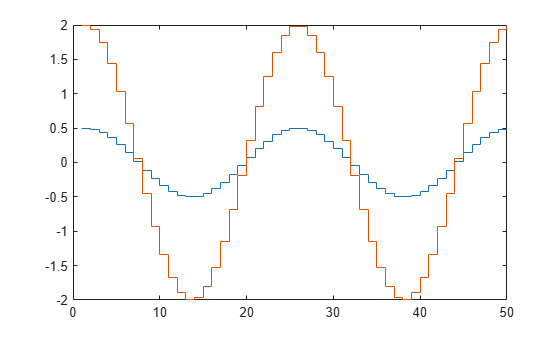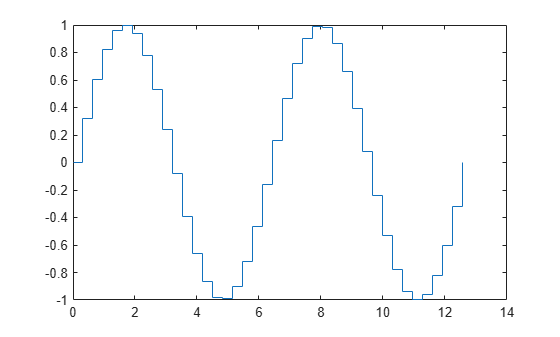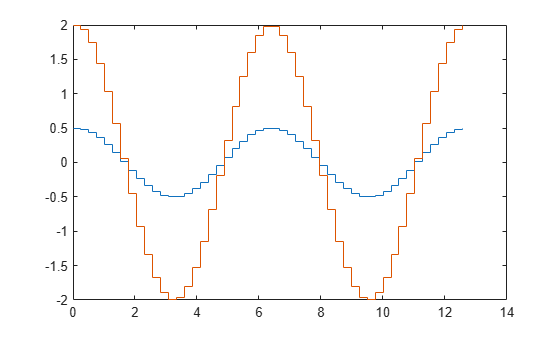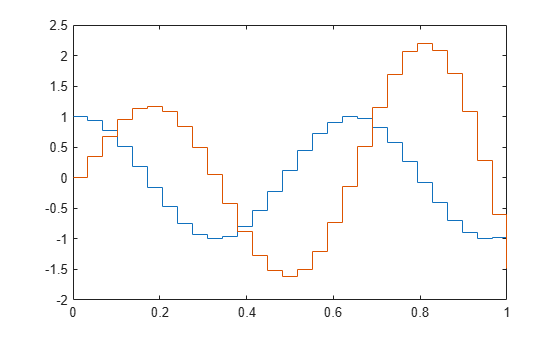Documentation

# stairs

Stairstep graph

## Syntax

``stairs(Y)``
``stairs(X,Y)``
``stairs(___,LineSpec)``
``stairs(___,Name,Value)``
``stairs(ax,___)``
``h = stairs(___)``
``````[xb,yb] = stairs(___)``````

## Description

example

````stairs(Y)` draws a stairstep graph of the elements in `Y`. If `Y` is a vector, then `stairs` draws one line.If `Y` is a matrix, then `stairs` draws one line per matrix column.```

example

````stairs(X,Y)` plots the elements in `Y` at the locations specified by `X`. The inputs `X` and `Y` must be vectors or matrices of the same size. Additionally, `X` can be a row or column vector and `Y` must be a matrix with `length(X)` rows. ```

example

````stairs(___,LineSpec)` specifies a line style, marker symbol, and color. For example, `':*r'` specifies a dotted red line with asterisk markers. Use this option with any of the input argument combinations in the previous syntaxes.```

example

````stairs(___,Name,Value)` modifies the stairstep chart using one or more name-value pair arguments. For example, `'Marker','o','MarkerSize',8` specifies 8 point circle markers.```

example

````stairs(ax,___)` plots into the axes specified by `ax` instead of into the current axes (`gca`). The option, `ax`, can precede any of the input argument combinations in the previous syntaxes.```

example

````h = stairs(___)` returns one or more `Stair` objects. Use `h` to make changes to properties of a specific `Stair` object after it is created. ```

example

``````[xb,yb] = stairs(___)``` does not create a plot, but returns matrices `xb` and `yb` of the same size, such that `plot(xb,yb)` plots the stairstep graph.```

## Examples

collapse all

Create a stairstep plot of sine evaluated at 40 equally spaced values between 0 and $4\pi$.

```X = linspace(0,4*pi,40); Y = sin(X); figure stairs(Y)```The length of `Y` automatically determines and generates the x-axis scale.

Create a stairstep plot of two cosine functions evaluated at 50 equally spaced values between 0 and $4\pi$.

```X = linspace(0,4*pi,50)'; Y = [0.5*cos(X), 2*cos(X)]; figure stairs(Y)```The number of rows in `Y` automatically determines and generates the x-axis scale.

Create a stairstep plot of a sine wave evaluated at equally spaced values between 0 and $4\pi$. Specify the set of x-values for the plot.

```X = linspace(0,4*pi,40); Y = sin(X); figure stairs(X,Y)```The entries in `Y` are plotted against the corresponding entries in `X`.

Create a stairstep plot of two cosine waves evaluated at equally spaced values between 0 and $4\pi$. Specify the set of x-values for the plot.

```X = linspace(0,4*pi,50)'; Y = [0.5*cos(X), 2*cos(X)]; figure stairs(X,Y)```The first vector input, `X`, determines the x-axis positions for both data series.

Create a stairstep plot of two sine waves evaluated at different values. Specify a unique set of x-values for plotting each data series.

```x1 = linspace(0,2*pi)'; x2 = linspace(0,pi)'; X = [x1,x2]; Y = [sin(5*x1),exp(x2).*sin(5*x2)]; figure stairs(X,Y)```Each column of `X` is plotted against the corresponding column of `Y`.

Create a stairstep plot and set the line style to a dot-dashed line, the marker symbol to circles, and the color to red.

```X = linspace(0,4*pi,20); Y = sin(X); figure stairs(Y, '-.or')```Create a stairstep plot and set the line width to 2, the marker symbols to diamonds, and the marker face color to cyan using `Name,Value` pair arguments.

```X = linspace(0,4*pi,20); Y = sin(X); figure stairs(Y,'LineWidth',2,'Marker','d','MarkerFaceColor','c')```Starting in R2019b, you can display a tiling of plots using the `tiledlayout` and `nexttile` functions. Call the `tiledlayout` function to create a 2-by-1 tiled chart layout. Call the `nexttile` function to create the axes objects `ax1` and `ax2`. Create separate stairstep plots in the axes by specifying the axes object as the first argument to `stairs`.

```x = linspace(0,2*pi); y1 = 5*sin(x); y2 = sin(5*x); tiledlayout(2,1) % Top plot ax1 = nexttile; stairs(ax1,x,y1) % Bottom plot ax2 = nexttile; stairs(ax2,x,y2)```Create a stairstep plot of two data series and return the two stair objects.

```X = linspace(0,1,30)'; Y = [cos(10*X), exp(X).*sin(10*X)]; h = stairs(X,Y);```Use small circle markers for the first data series. Use magenta filled circles for the second series. Starting in R2014b, you can use dot notation to set properties. If you are using an earlier release, use the `set` function instead.

```h(1).Marker = 'o'; h(1).MarkerSize = 4; h(2).Marker = 'o'; h(2).MarkerFaceColor = 'm';```Evaluate two cosine functions at 50 equally spaced values between 0 and $4\pi$ and create a stairstep plot using `plot`.

```X = linspace(0,4*pi,50)'; Y = [0.5*cos(X), 2*cos(X)]; [xb,yb] = stairs(X,Y);```

`stairs` returns two matrices of the same size, `xb` and `yb`, but no plot.

Use `plot` to create the stairstep plot with `xb` and `yb`.

```figure plot(xb,yb)```## Input Arguments

collapse all

y values, specified as a vector or matrix. When `Y` is a vector, `stairs` creates one stair object. When `Y` is a matrix, `stairs` draws one line per matrix column and creates a separate stair object for each column.

Data Types: `single` | `double` | `int8` | `int16` | `int32` | `int64` | `uint8` | `uint16` | `uint32` | `uint64` | `categorical` | `datetime` | `duration`

x values, specified as a vector or matrix. When `Y` is a vector, `X` must be a vector of the same size. When `Y` is a matrix, `X` must be a matrix of the same size, or a vector whose length equals the number of rows in `Y`.

Data Types: `single` | `double` | `int8` | `int16` | `int32` | `int64` | `uint8` | `uint16` | `uint32` | `uint64` | `categorical` | `datetime` | `duration`

Line style, marker symbol, and color, specified as a character vector or string. For more information on line style, marker symbol, and color options see `LineSpec`.

Example: `':*r'`

`Axes` object. If you do not specify the axes, then `stairs` plots into the current axes.

### Name-Value Pair Arguments

Specify optional comma-separated pairs of `Name,Value` arguments. `Name` is the argument name and `Value` is the corresponding value. `Name` must appear inside quotes. You can specify several name and value pair arguments in any order as `Name1,Value1,...,NameN,ValueN`.

Example: `'Marker','s','MarkerFaceColor','red'` plots the stairstep graph with red square markers.

The properties listed here are only a subset. For a complete list, see Stair Properties.

Line style, specified as one of the options listed in this table.

Line StyleDescriptionResulting Line
`'-'`Solid line`'--'`Dashed line`':'`Dotted line`'-.'`Dash-dotted line`'none'`No lineNo line

Line width, specified as a positive value in points, where 1 point = 1/72 of an inch. If the line has markers, then the line width also affects the marker edges.

Line color, specified as an RGB triplet, a hexadecimal color code, a color name, or a short name.

For a custom color, specify an RGB triplet or a hexadecimal color code.

• An RGB triplet is a three-element row vector whose elements specify the intensities of the red, green, and blue components of the color. The intensities must be in the range `[0,1]`; for example, ```[0.4 0.6 0.7]```.

• A hexadecimal color code is a character vector or a string scalar that starts with a hash symbol (`#`) followed by three or six hexadecimal digits, which can range from `0` to `F`. The values are not case sensitive. Thus, the color codes `'#FF8800'`, `'#ff8800'`, `'#F80'`, and `'#f80'` are equivalent.

Alternatively, you can specify some common colors by name. This table lists the named color options, the equivalent RGB triplets, and hexadecimal color codes.

Color NameShort NameRGB TripletHexadecimal Color CodeAppearance
`'red'``'r'``[1 0 0]``'#FF0000'``'green'``'g'``[0 1 0]``'#00FF00'``'blue'``'b'``[0 0 1]``'#0000FF'``'cyan'` `'c'``[0 1 1]``'#00FFFF'``'magenta'``'m'``[1 0 1]``'#FF00FF'``'yellow'``'y'``[1 1 0]``'#FFFF00'``'black'``'k'``[0 0 0]``'#000000'``'white'``'w'``[1 1 1]``'#FFFFFF'``'none'`Not applicableNot applicableNot applicableNo color

Here are the RGB triplets and hexadecimal color codes for the default colors MATLAB® uses in many types of plots.

RGB TripletHexadecimal Color CodeAppearance
`[0 0.4470 0.7410]``'#0072BD'``[0.8500 0.3250 0.0980]``'#D95319'``[0.9290 0.6940 0.1250]``'#EDB120'``[0.4940 0.1840 0.5560]``'#7E2F8E'``[0.4660 0.6740 0.1880]``'#77AC30'``[0.3010 0.7450 0.9330]``'#4DBEEE'``[0.6350 0.0780 0.1840]``'#A2142F'`Example: `'blue'`

Example: ```[0 0 1]```

Example: `'#0000FF'`

Marker symbol, specified as one of the values listed in this table. By default, the object does not display markers. Specifying a marker symbol adds markers at each data point or vertex.

ValueDescription
`'o'`Circle
`'+'`Plus sign
`'*'`Asterisk
`'.'`Point
`'x'`Cross
`'square'` or `'s'`Square
`'diamond'` or `'d'`Diamond
`'^'`Upward-pointing triangle
`'v'`Downward-pointing triangle
`'>'`Right-pointing triangle
`'<'`Left-pointing triangle
`'pentagram'` or `'p'`Five-pointed star (pentagram)
`'hexagram'` or `'h'`Six-pointed star (hexagram)
`'none'`No markers

Marker size, specified as a positive value in points, where 1 point = 1/72 of an inch.

Marker outline color, specified as `'auto'`, an RGB triplet, a hexadecimal color code, a color name, or a short name. The default value of `'auto'` uses the same color as the `Color` property.

For a custom color, specify an RGB triplet or a hexadecimal color code.

• An RGB triplet is a three-element row vector whose elements specify the intensities of the red, green, and blue components of the color. The intensities must be in the range `[0,1]`; for example, ```[0.4 0.6 0.7]```.

• A hexadecimal color code is a character vector or a string scalar that starts with a hash symbol (`#`) followed by three or six hexadecimal digits, which can range from `0` to `F`. The values are not case sensitive. Thus, the color codes `'#FF8800'`, `'#ff8800'`, `'#F80'`, and `'#f80'` are equivalent.

Alternatively, you can specify some common colors by name. This table lists the named color options, the equivalent RGB triplets, and hexadecimal color codes.

Color NameShort NameRGB TripletHexadecimal Color CodeAppearance
`'red'``'r'``[1 0 0]``'#FF0000'``'green'``'g'``[0 1 0]``'#00FF00'``'blue'``'b'``[0 0 1]``'#0000FF'``'cyan'` `'c'``[0 1 1]``'#00FFFF'``'magenta'``'m'``[1 0 1]``'#FF00FF'``'yellow'``'y'``[1 1 0]``'#FFFF00'``'black'``'k'``[0 0 0]``'#000000'``'white'``'w'``[1 1 1]``'#FFFFFF'``'none'`Not applicableNot applicableNot applicableNo color

Here are the RGB triplets and hexadecimal color codes for the default colors MATLAB uses in many types of plots.

RGB TripletHexadecimal Color CodeAppearance
`[0 0.4470 0.7410]``'#0072BD'``[0.8500 0.3250 0.0980]``'#D95319'``[0.9290 0.6940 0.1250]``'#EDB120'``[0.4940 0.1840 0.5560]``'#7E2F8E'``[0.4660 0.6740 0.1880]``'#77AC30'``[0.3010 0.7450 0.9330]``'#4DBEEE'``[0.6350 0.0780 0.1840]``'#A2142F'`Marker fill color, specified as `'auto'`, an RGB triplet, a hexadecimal color code, a color name, or a short name. The `'auto'` option uses the same color as the `Color` property of the parent axes. If you specify `'auto'` and the axes plot box is invisible, the marker fill color is the color of the figure.

For a custom color, specify an RGB triplet or a hexadecimal color code.

• An RGB triplet is a three-element row vector whose elements specify the intensities of the red, green, and blue components of the color. The intensities must be in the range `[0,1]`; for example, ```[0.4 0.6 0.7]```.

• A hexadecimal color code is a character vector or a string scalar that starts with a hash symbol (`#`) followed by three or six hexadecimal digits, which can range from `0` to `F`. The values are not case sensitive. Thus, the color codes `'#FF8800'`, `'#ff8800'`, `'#F80'`, and `'#f80'` are equivalent.

Alternatively, you can specify some common colors by name. This table lists the named color options, the equivalent RGB triplets, and hexadecimal color codes.

Color NameShort NameRGB TripletHexadecimal Color CodeAppearance
`'red'``'r'``[1 0 0]``'#FF0000'``'green'``'g'``[0 1 0]``'#00FF00'``'blue'``'b'``[0 0 1]``'#0000FF'``'cyan'` `'c'``[0 1 1]``'#00FFFF'``'magenta'``'m'``[1 0 1]``'#FF00FF'``'yellow'``'y'``[1 1 0]``'#FFFF00'``'black'``'k'``[0 0 0]``'#000000'``'white'``'w'``[1 1 1]``'#FFFFFF'``'none'`Not applicableNot applicableNot applicableNo color

Here are the RGB triplets and hexadecimal color codes for the default colors MATLAB uses in many types of plots.

RGB TripletHexadecimal Color CodeAppearance
`[0 0.4470 0.7410]``'#0072BD'``[0.8500 0.3250 0.0980]``'#D95319'``[0.9290 0.6940 0.1250]``'#EDB120'``[0.4940 0.1840 0.5560]``'#7E2F8E'``[0.4660 0.6740 0.1880]``'#77AC30'``[0.3010 0.7450 0.9330]``'#4DBEEE'``[0.6350 0.0780 0.1840]``'#A2142F'`## Output Arguments

collapse all

`Stair` objects. These are unique identifiers, which you can use to query and modify the properties of a specific `Stair` object after it is created.

x values for use with `plot`, returned as a vector or matrix. `xb` contains the appropriate values such that `plot(xb,yb)` creates the stairstep graph.

y values for use with `plot`, returned as a vector or matrix. `yb` contains the appropriate values such that `plot(xb,yb)` creates the stairstep graph.